We will introduce two substantial features of our approach. To do so, let us assume an optical element that may be accurately simulated by the BPM. The typical representing device can be the four-port coupler shown on the first figure in this paper. Our aim is rather more complex and general study of the advanced photonic circuits. Let us recognize the coupler as a device connecting a certain number of input and output ports. In other words, let the BPM be the technique yielding the response at the output ports if given modal fields were introduced into the input port. The star coupler is an example of a more advanced BPM element (see Figure 6).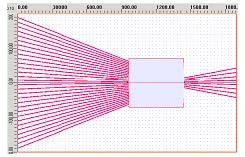Figure 6: Star coupler

We can see quite a number of the input ports (on the left hand side) and some number of the output ports (the right hand side). Actually, we do not need to care much what happens inside the simulation window. This has been determined by the BPM and what we actually wish to collect is the pertinent data at the outputs. Since we are dealing with the optical circuits we would need some kind of information carrying the transfer of the power among the input and the output ports accompanied with the phase change. The principle of this sub-circuit analysis is very simple and needs just a negligible action by a user. Having a layout created under the OptiBPM, like the four- port coupler (i.e. 2 input ports and 2 output ports) or the star coupler (thus generally designed as N input and M output ports), one can click the icon called Scattering data script icon seen below).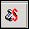What happens then is fully automated by OptiBPM. Namely, the scattering data script
icon generates the script yielding the scanning through a given interval of wavelengths. Running the simulation thereafter, we will receive the S-data file fully describing the optical response of the BPM sub-circuit under study. Let us show how it really works in the following.

Any suitable layout for our approach can be schematically depicted as shown in Figure 7. Such layouts consist of few basic items. Firstly, there is a given rectangular simulation window (understood as the wafer in the OptiBPM). The propagation direction BPM propagation direction is stated from the left to the right. Therefore, we have M input ports on the left and N output ports on the right. Both types of ports are supposed to have the light in a modal configuration. In addition, they may be arbitrarily tilted and each input/output waveguide may be of a different geometry. The only one condition is the waveguides must carry the light in the fundamental modal field. These ports can be understood as the connectors, it will be mentioned later in the final implementation part.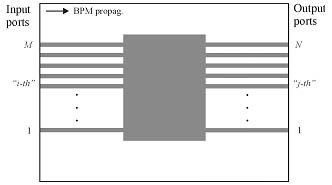Figure 7: Scattering Data layout schematic

We would now like to know how is the entire device (the schematics show the MMI coupler) response to the introduced modal field. The simulation run under the S-data script does the following. The simulation starts with the propagation of a pertinent modal field from the first input waveguide. Whatever happens inside the simulation window, we expect some modal field at each output waveguide. The second step of the scripted loop is the response from the second waveguide up to the M -th one. All this is performed for several user defined number of wavelengths, as seen in the scripting loop.

We may describe the procedure mathematically. Let us begin with the trivial four-port coupler (see Figure 1). Having known the input fields a1  and a2 , we may construct the schematic matrix S yielding the desired information at the output ports b1  and b2 (note there is some analogy with the classical “scattering matrix”, however we use a reduced form of it, hence it is called S-data matrix through the text). The four-port coupler is then characterized by the following relation:Equation 1 can be easily described in an oral form. The element S11  is responsible for a response from the first input waveguide into the first output waveguide. Similarly, the matrix element S12 relates to the response in the second output waveguide when the light was introduced into the first input waveguide. The meaning of the other matrix elements is defined in analogous way. Note, the elements are complex numbers and they consist of real and imaginary parts. Equation 1 has to be repeatedly determined for each wavelength run. The relations among the input and output ports takes the general form (for one particular wavelength)We use the following relations to obtain the desired S-data elements. We are searching for a response on a unitary input modal field (unitary means the unit amplitude and zero phase additional advance of the modal filed). We have to use the following approximation, because it would be somehow cumbersome to transport a complete information carrying the output field distribution yielded from the BPM simulation. Concerning the relative power presence at the output waveguide, we thus compare the actual final BPM field obtained after the simulation at each output waveguide with that one being theoretically present if the single straight waveguide light propagation considered for the pertinent output waveguide. This can be easily done by well-known power overlap integralwhere one field is the BPM one, while the second is the modal field distribution of output waveguide (the asterisk means the complex conjugated field). The denominator, consisting of the product of two integrals, ensures the normalization of the overlap integral from 0 to 1. Ω determines the integration region.

The response with respect to the cumulated phase delay is then given by (both real and imaginary part)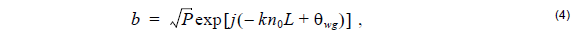where =  2π ⁄ λ , n0  is the reference index, L is the propagation length. Finally, θwg  is an additional angle related to the BPM propagation (this can be observed from the analyzer). Sometimes in one BPM calculation more than one reference index will be used. There may be regions having different reference indices. If more then one region appears in the layout, we have to characterize these regions separately by particular lengths and reference indices and the phase delay term kn0L in Equation 4 must be replaced accordingly as shown schematically in Equation 5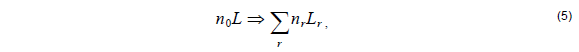with r being the number of regions in the layout. In other words Equation 4 simply adds the phase shift due to the optical length of the wafer. Since the responses were sought for the unit input modal fields, the collected values of b for each combination (input/output/wavelength) created according to Equation 2 the S-data matrix Sij elements. The exported file is a text file with an ‘.s‘ extension. The first line contains two integer values: the number of inputs and the number of outputs. All the subsequent lines represent an individual scattering data matrix for a single wavelength. They contain the wavelength in microns and all the complex values of the amplitude transmission between each input and output port. The total number of elements in each row is the number of the inputs times the number of the outputs times 2 (real and imaginary parts) plus one (wavelength). These lines appear such that the wavelength is in increasing order.

## Central wavelength approximation

We can, however, employ a more approximate solution which is much faster to compute. Concerning the wavelength interval, we are mostly dealing with a very small vicinity of some central wavelength, say. To save more simulation time, we can run the simulation just for this wavelength to obtain the one set of matrix elements. Let us call the matrix Sc  (the *.s file will then be just one line with the headings). In most practical applications, the weighted power distribution coming from the power overlap integral in an output port varies by a negligible amount. The phase change may be crucial, on the other hand. We can then approximately derive the phase change with respect to the wavelength. We can approximately write in the vicinity of a central wavelength λc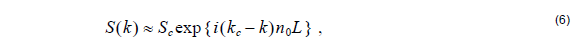with kc = 2π ⁄ λc , while = 2π ⁄ λ where λ is the actual wavelength. The values Sc  are the S-data obtained after the ordinary simulation for the central wavelength. In conclusion, we may need just one wavelength simulation to describe the device optical response. The limitation of the central wavelength approximation is obvious.

The accuracy is decreased with increasing propagation length as well as with the broader wavelength interval. The approximation will be also suitable for lower values of the reference refractive index.